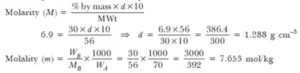# Solutions Chemistry Class 12 Chapter Solution Test Paper – 2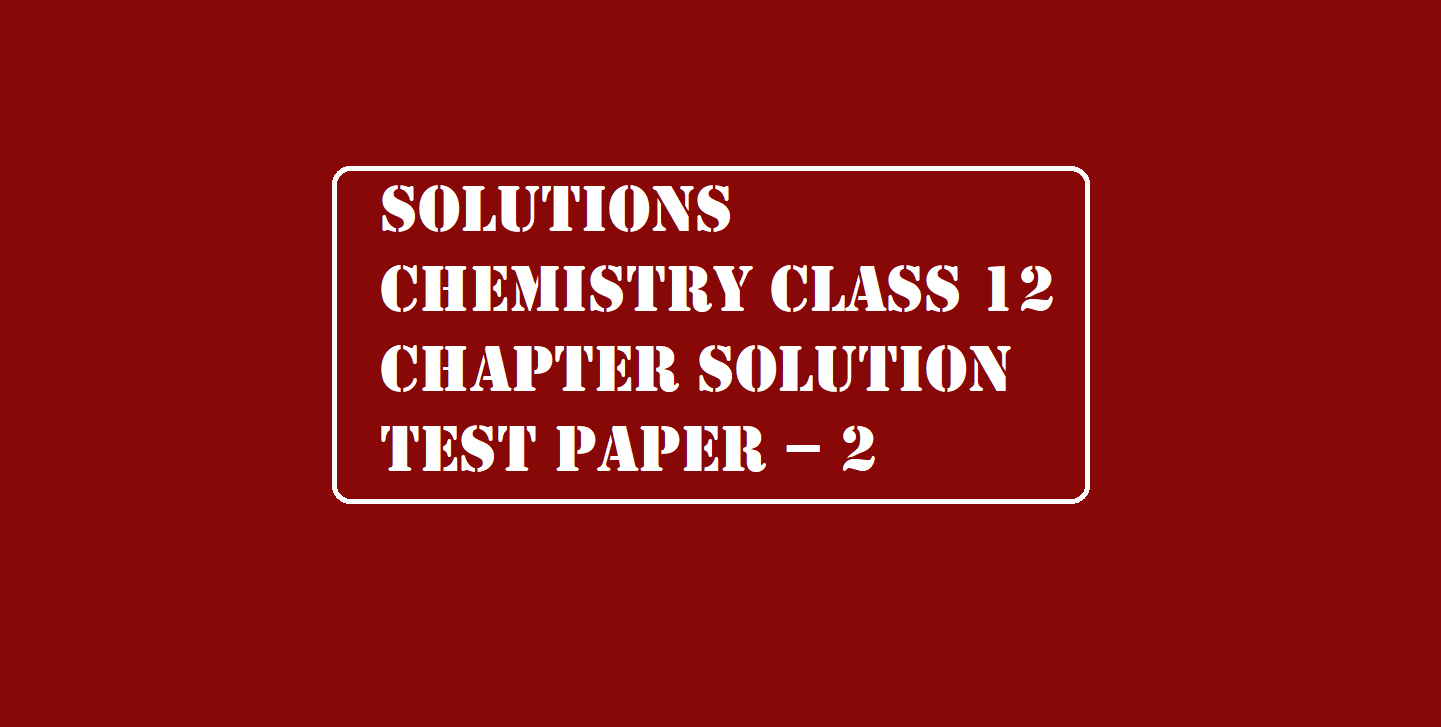# Solutions Chemistry Class 12 Chapter Solution Test Paper – 2

Solutions Class 12 Chapter Solution Test Paper

Ans 1.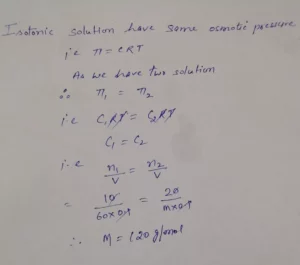Ans 2. The solute is 50% dissociated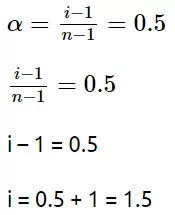Ans 3.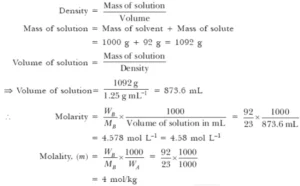Ans 4.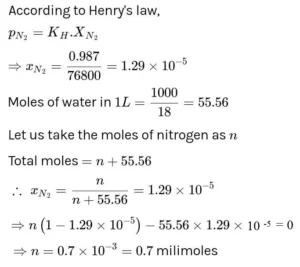Ans 5. According to Raoult’s law,
(p0 – p) / p0 = (w × M) / (m × W)
p0 → is Vapour pressure of pure water
p → is vapour pressure of the solvent
w → is weight of solute
m → is the molecular weight of solute
W → is the molecular weight of solvent
(32 – 31.84) / 32 = (10 × 18) / (m × 200)
m = 180 g/mol.

Solutions Class 12 Chapter Solution Test Paper

Ans 6.  p0 = 0.850 bar = Vapour pressure of pure benzene.
p = 0.845 bar = Vapour pressure of solution.
w1 = 39 g = Mass of benzene
w2 = 78 g = Mass of solute
M1 = 78 g/mol = Molar mass of benzene.
M2 = Molar mass of solute.
(p0 – p)/p = w2 × M1 / w1 × M2
(p0 – p) = 0.850 – 0.845 = 0.005 bar
w2 × M1 = 0.5 × 78 = 39
w1 × M2 = 39 × M2
0.005 = 39/39 × M2
0.005/0.850 = 1/M2
M2 = 1 × 0.850 / 0.005 = 170 g/mol

Ans 7.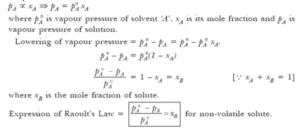Ans 8. Moles of glucose = 18 g/ 180 g mol–1 = 0.1 mol
No of kg of solvent = 1 kg
therefore, molality of glucose solution = 0.1 mol kg-1
For water, change in boiling point
ΔTb = Kb × m = 0.52 K kg mol–1 × 0.1 mol kg–1 = 0.052 K
Since water boils at 373.15 K at 1.013 bar pressure.
The boiling point of solution will be 373.15 + 0.052 = 373.202 K.

Ans 9. 80.2 g of water contains = 0.520 g
1000 g of water contains = 0.52 × 1000/80.2 = 6.484 g of Glucose.
No. of moles of Glucose = 6.484/180 = 0.036
increase in boiling point of water = Kb × m,
where’s Kb = 0.52, m = 0.036.
So ∆Tb = 0.52 × 0.036 = 0.0187
So boiling point of Solution
= 100° + 0.0187° = 100.0187°C
= 273 + 100.0187 = 300.0187 K

Ans 10.  Kb for benzene = 2.53 K KJ/mole
Mass of solute = W2 = 1.8 g = 1.8 × 10-3 kg
Mass of Solvent = W1 = 90g = 90 × 10-3
Boiling point of solution = Tb = 354.11 K
Boiling point of pure solvent = Tb’ = 353.23 K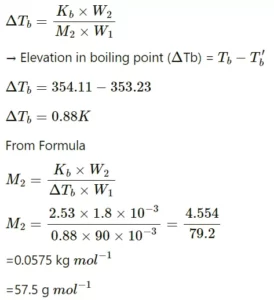Ans 11. Given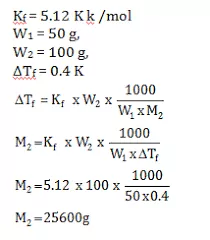Ans 12.  We know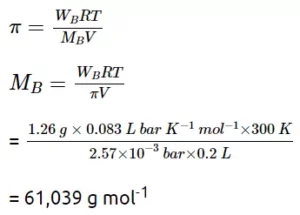Ans 13. CCl3​COOH → CCl3​COO+ H+
ΔTb​ = (100.18 + 273) − (100 − 273) = 0.18K
Molality is 1.
Hence, ΔTb​ = iKb​m = iKb ​× 1
i = ΔTb / Kb
i = 0.18 / 0.512
i = 0.351

Ans 14. We know
ΔTf = i Kf​ m
2.93 = 1.86 × 5.61 × i
i = 1.86 × 5.61 / 2.93
i = 0.28

Ans 15. We know
ΔTf = i Kf​ m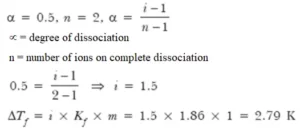Freezing point of solution = 273 – 2.79 = 270.21 K

Ans 16. 1 molar solution means that 1 gram mole of KCI is dissolved in one litre of the solution.
Mass of 1L of solution = V × d = 1000 × 1.04 = 1040 g
Mass of one mole KCI = 39 + 35.5 = 74.5 g
Mass of solvent =1040 – 74.5 = 965.5 g = 0.965 kg
Molality of solution (m) = No. of moles of solute / Mass of solvent in kg
= 1/ 0.965 mol/kg = 1.0357 m
KCl dissociates as: KCl → K+ + Cl
∴ Number of particles after dissociation = 2
∴ van’t Hoff factor, i = 2
Now ΔTb = i × Kb × m = 2 × 0.52 × 1.0357 = 1.078 C
∴ Boiling point of the solution = 100 + 1.078 = 101.078 C

Ans 17. As NaCl undergoes complete dissociation
NaCl → Na+ + Cl
One mole of NaCl will give 2 particle and the value of i will be equal to 2.
ΔTf = i kf m
Kf = 1.86 K m−1,
ΔTf = 3K, i=2
∴ 3 = 2 × 1.86 × m
∴ m = 0.806 mol/kg
Amount of NaCl = 0.806 × 58.5 = 47.151 = 0.806 × 58.5 = 47.151 g/kg

Ans 18.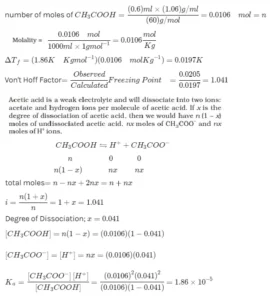Ans 19.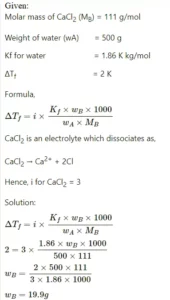Ans 20.